# RD Sharma Solutions for Class 6 Maths Chapter 3: Whole Numbers Exercise 3.1

Exercise 3.1 of Chapter 3 covers major concepts like natural numbers and whole numbers along with their properties which are important from the exam point of view. RD Sharma Solutions Class 6 includes the textbook problems based on CBSE syllabus to help students obtain a better academic score. The students can gain a better hold on the concepts by downloading RD Sharma Solutions for Class 6 Maths Chapter 3 Whole Numbers Exercise 3.1 PDF which are provided here.

## RD Sharma Solutions for Class 6 Maths Chapter 3: Whole Numbers Exercise 3.1 Download PDF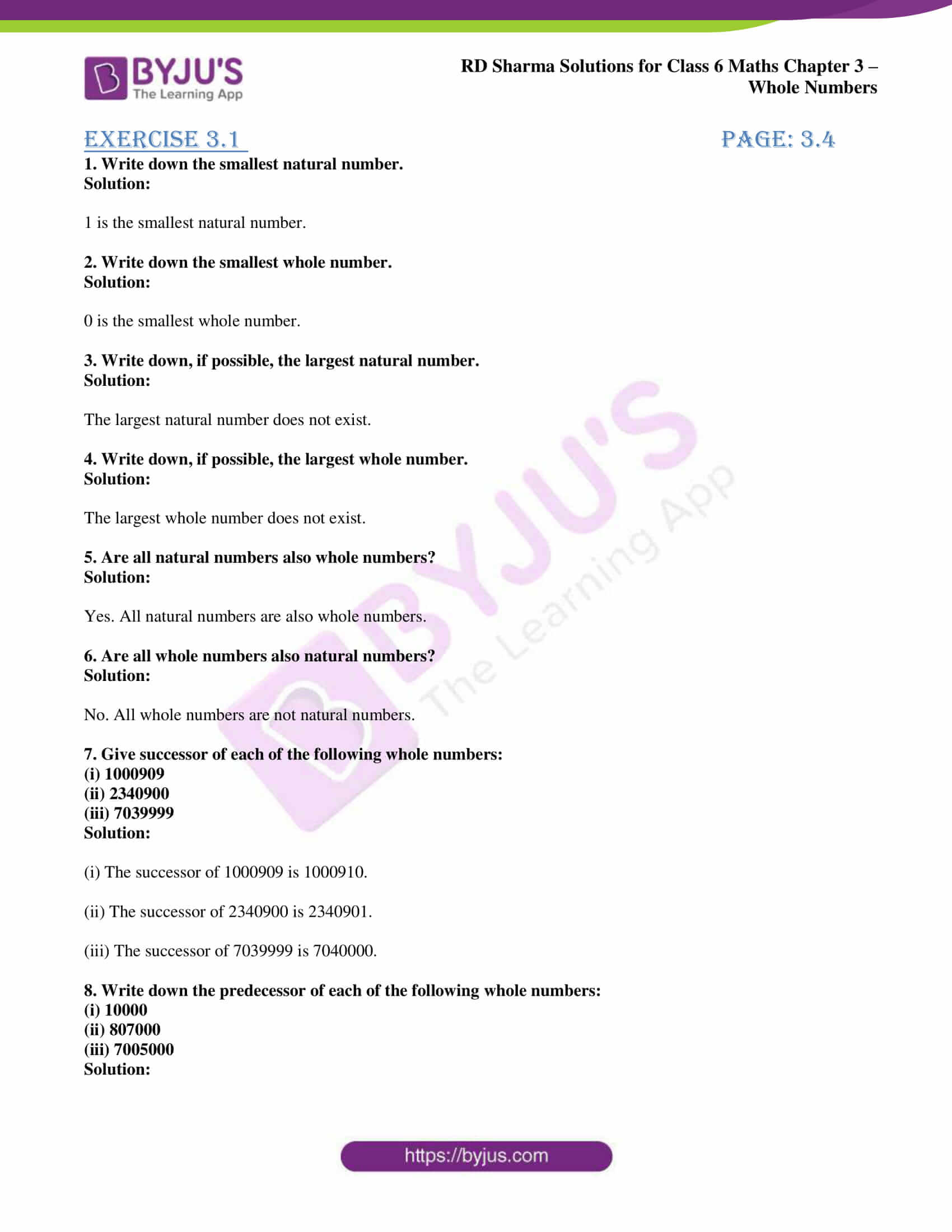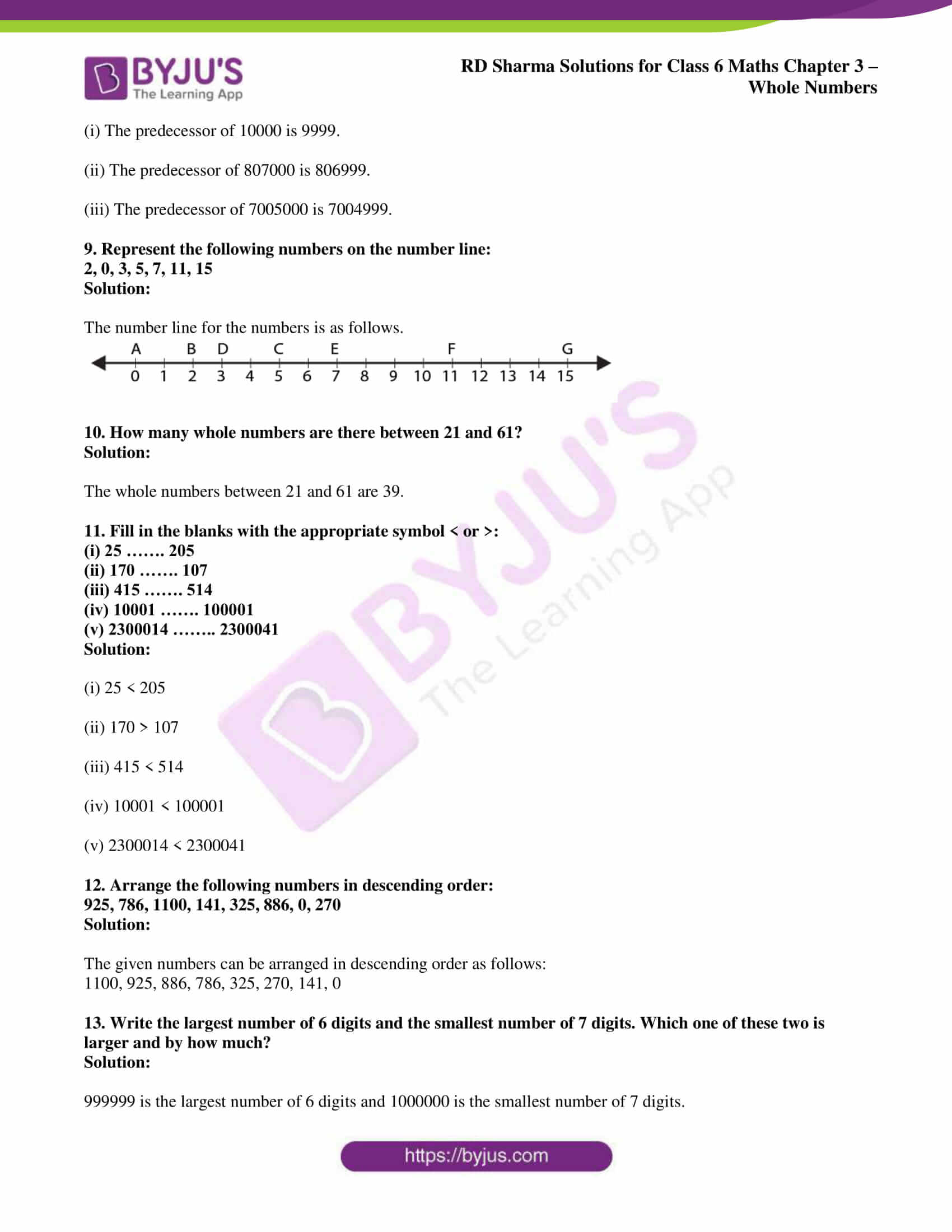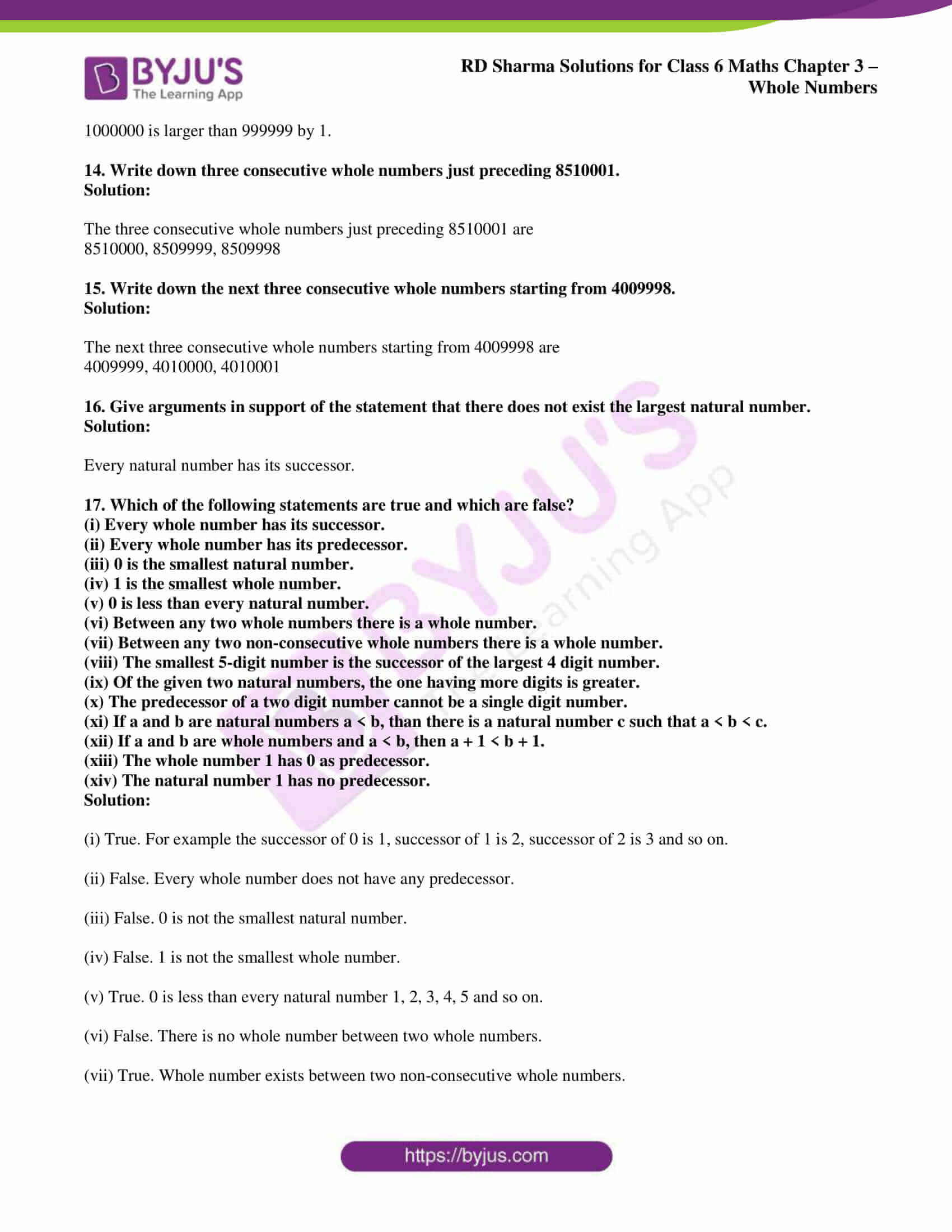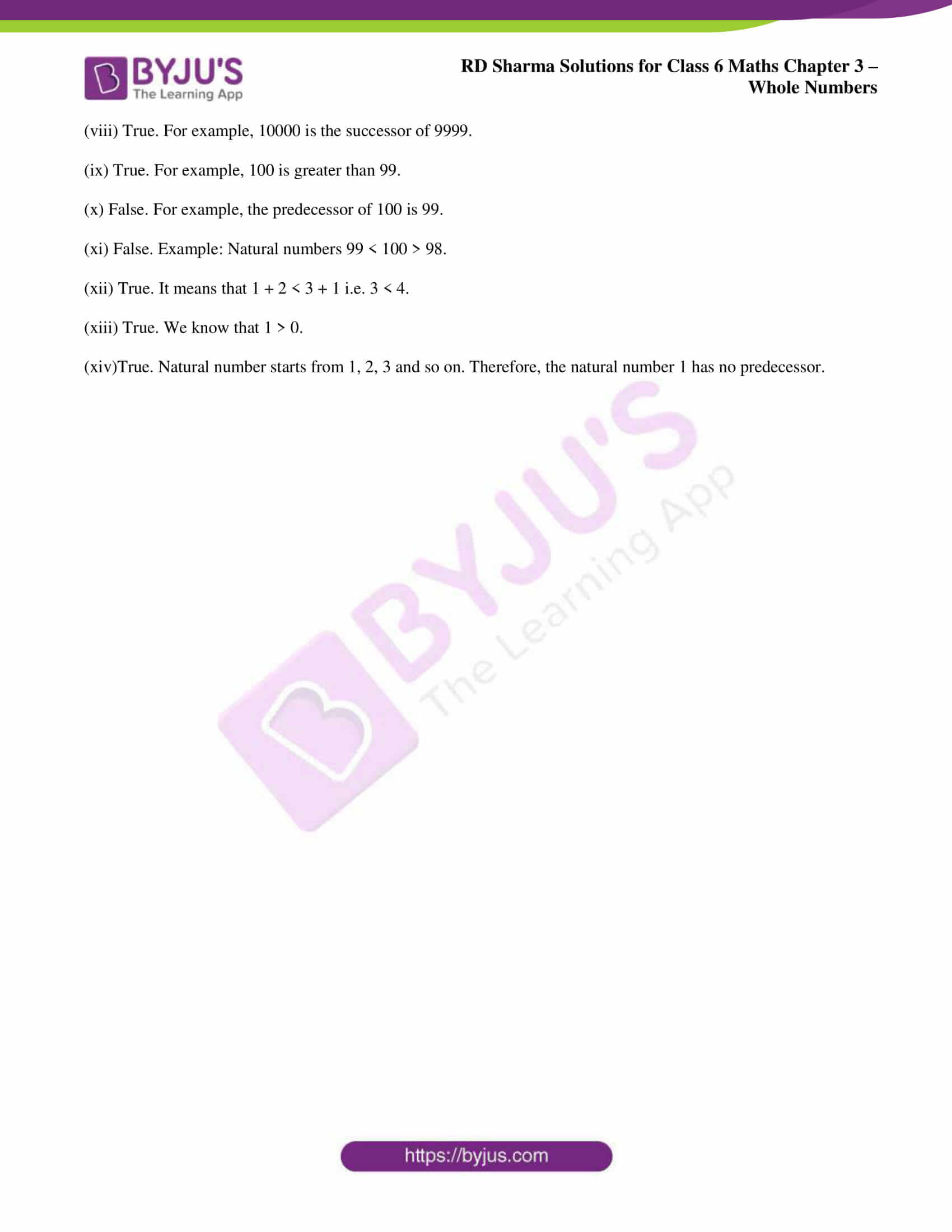### Access answers to Maths RD Sharma Solutions for Class 6 Chapter 3: Whole Numbers Exercise 3.1

1. Write down the smallest natural number.

Solution:

1 is the smallest natural number.

2. Write down the smallest whole number.

Solution:

0 is the smallest whole number.

3. Write down, if possible, the largest natural number.

Solution:

The largest natural number does not exist.

4. Write down, if possible, the largest whole number.

Solution:

The largest whole number does not exist.

5. Are all natural numbers also whole numbers?

Solution:

Yes. All natural numbers are also whole numbers.

6. Are all whole numbers also natural numbers?

Solution:

No. All whole numbers are not natural numbers.

7. Give successor of each of the following whole numbers:

(i) 1000909

(ii) 2340900

(iii) 7039999

Solution:

(i) The successor of 1000909 is 1000910.

(ii) The successor of 2340900 is 2340901.

(iii) The successor of 7039999 is 7040000.

8. Write down the predecessor of each of the following whole numbers:

(i) 10000

(ii) 807000

(iii) 7005000

Solution:

(i) The predecessor of 10000 is 9999.

(ii) The predecessor of 807000 is 806999.

(iii) The predecessor of 7005000 is 7004999.

9. Represent the following numbers on the number line:

2, 0, 3, 5, 7, 11, 15

Solution:

The number line for the numbers is as follows.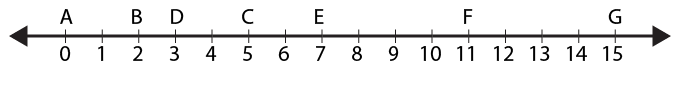10. How many whole numbers are there between 21 and 61?

Solution:

The whole numbers between 21 and 61 are 39.

11. Fill in the blanks with the appropriate symbol < or >:

(i) 25 ……. 205

(ii) 170 ……. 107

(iii) 415 ……. 514

(iv) 10001 ……. 100001

(v) 2300014 …….. 2300041

Solution:

(i) 25 < 205

(ii) 170 > 107

(iii) 415 < 514

(iv) 10001 < 100001

(v) 2300014 < 2300041

12. Arrange the following numbers in descending order:

925, 786, 1100, 141, 325, 886, 0, 270

Solution:

The given numbers can be arranged in descending order as follows:

1100, 925, 886, 786, 325, 270, 141, 0

13. Write the largest number of 6 digits and the smallest number of 7 digits. Which one of these two is larger and by how much?

Solution:

999999 is the largest number of 6 digits and 1000000 is the smallest number of 7 digits.

1000000 is larger than 999999 by 1.

14. Write down three consecutive whole numbers just preceding 8510001.

Solution:

The three consecutive whole numbers just preceding 8510001 are

8510000, 8509999, 8509998

15. Write down the next three consecutive whole numbers starting from 4009998.

Solution:

The next three consecutive whole numbers starting from 4009998 are

4009999, 4010000, 4010001

16. Give arguments in support of the statement that there does not exist the largest natural number.

Solution:

Every natural number has its successor.

17. Which of the following statements are true and which are false?

(i) Every whole number has its successor.

(ii) Every whole number has its predecessor.

(iii) 0 is the smallest natural number.

(iv) 1 is the smallest whole number.

(v) 0 is less than every natural number.

(vi) Between any two whole numbers there is a whole number.

(vii) Between any two non-consecutive whole numbers there is a whole number.

(viii) The smallest 5-digit number is the successor of the largest 4 digit number.

(ix) Of the given two natural numbers, the one having more digits is greater.

(x) The predecessor of a two digit number cannot be a single digit number.

(xi) If a and b are natural numbers a < b, than there is a natural number c such that a < b < c.

(xii) If a and b are whole numbers and a < b, then a + 1 < b + 1.

(xiii) The whole number 1 has 0 as predecessor.

(xiv) The natural number 1 has no predecessor.

Solution:

(i) True. For example the successor of 0 is 1, successor of 1 is 2, successor of 2 is 3 and so on.

(ii) False. Every whole number does not have any predecessor.

(iii) False. 0 is not the smallest natural number.

(iv) False. 1 is not the smallest whole number.

(v) True. 0 is less than every natural number 1, 2, 3, 4, 5 and so on.

(vi) False. There is no whole number between two whole numbers.

(vii) True. Whole number exists between two non-consecutive whole numbers.

(viii) True. For example, 10000 is the successor of 9999.

(ix) True. For example, 100 is greater than 99.

(x) False. For example, the predecessor of 100 is 99.

(xi) False. Example: Natural numbers 99 < 100 > 98.

(xii) True. It means that 1 + 2 < 3 + 1 i.e. 3 < 4.

(xiii) True. We know that 1 > 0.

(xiv)True. Natural number starts from 1, 2, 3 and so on. Therefore, the natural number 1 has no predecessor.